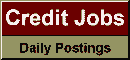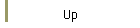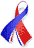DefaultRisk.com the web's biggest credit risk modeling resource.Export citation to:- HTML- Text (plain)- BibTeX- RIS- ReDIF

On Approximation of Functions by Exponential Sums

by Gregory Beylkin of the University of Colorado, and
Lucas Monzón of the University of Colorado

March 2005

Abstract: We introduce a new approach, and associated algorithms, for the efficient approximation of functions and sequences by short linear combinations of exponential functions with complex-valued exponents and coefficients. These approximations are obtained for a finite but arbitrary accuracy and typically have significantly fewer terms than Fourier representations. We present several examples of these approximations and discuss applications to fast algorithms. In particular, we show how to obtain a short separated representation (sum of products of one dimensional functions) of certain multi-dimensional Green's functions.

Keywords: Exponential sums, Hankel matrices, Prony's method, Separated representations.

Published in: Applied and Computational Harmonic Analysis, Vol. 19, No. 1, (March 2005), pp. 17-48.

Books Referenced in this paper:  (what is this?)